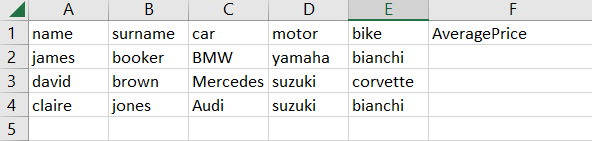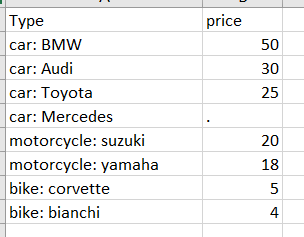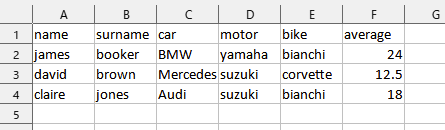# Find the average of each row

Hi everyone,

I have 2 tables like belowOne table has the prices of vehicles the other has the people’s information. I want to be able to calculate the average price needed to given for each person and write it to the average price column.

Side note: for example Mercedes has no price given so I don’t want it to be included in the calculation.

Any ideas?

@berkaykor

Inside the “For Each” activity, add a “Assign” activity and create a new variable called “sum” of type “Double”, and use the following expression to add the value of each cell in the current row to the sum: `sum = sum + CDbl(row(column)) `

After the inner loop completes, add a “Assign” activity to calculate the average by dividing the sum by the number of columns, and store the result in a variable called “average”. Use the following expression: `average = sum / row.ItemArray.Length `

but how would it know how much is each item? and there is more than 1 column to take the average of the row

I want to be able to find the average price of the vehicles for each person.

For example: James Booker, BMW, yamaha and bianchi. I check the price table and see car: BMW is 50 motorcycle: yamaha is 18 and bike: bianchi is 4 average is 24.

Hi,

Hope the following sample helps you.

``````dict =dt2.AsEnumerable.Where(Function(r) Int32.TryParse(r("price").ToString,New Int32)).ToDictionary(Function(r) r("Type").ToString,Function(r) Int32.Parse(r("price").ToString))
``````

then

``````dt1 = dt1.AsEnumerable.Select(Function(r) dt1.Clone.LoadDataRow({r(0),r(1),r(2),r(3),r(4),r.ItemArray.Select(Function(o,i) if(dict.ContainsKey(dt1.Columns(i).ColumnName.Replace("motor","motorcycle")+": "+o.ToString),dict(dt1.Columns(i).ColumnName.Replace("motor","motorcycle")+": "+o.ToString).ToString,"")).Where(Function(s) not String.IsNullOrEmpty(s)).Average(Function(s) CDbl(s))},False)).CopyToDataTable
``````

ResultSample

Sample20230503-2aL.zip (3.3 KB)

Regards,

1 Like

Hi @berkaykor ,

Take a look at this thread. It helps you.

Thank you
Balamurugan.S

I cannot use outsourced packagesHi Yoichi,

Yes your code works, but is there a way to not use Linq for this problem? I have to keep it as low code as possible.

Wow, thank you so much.

I am getting the following error on Assign: Cannot create an L-value from the given expression with property ‘set_Item’ because the target object is null.

when im trying to parse and add to the dictionary.

Sorry I forgot to initialize the dictionary thats why.